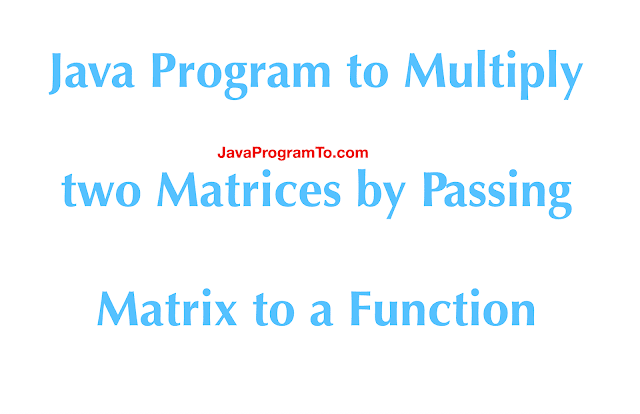## \$show=/label

A quick and practical programming guide to multiply two matrix using a user defined a function. This function has the core logic for multiplication.

## 1. Overview

In this tutorial, you'll learn how to write the function for matrix multiplication. Let us pass the input and result matrices to this method and will run the core logic to multiply. Finally, the output is stored in the result array.

In the previous article, shown how to multiply two matrices without using function.

Example:

Matrix 1 order: 2 X 3

Matrix 2 order: 3 X 2

Result matrix order: 2 X 2

key note in the matrix multiplication is always matrix 1 columns size and matrix two row size must be equal. Otherwise matrix multiplication is not possible.

## 2. Matrix Multiplication Traversal

First, let us take a generic example with the 2 X 2 size two matrices as below.

Matrix M1 : [A11, A12        Matrix M2 : [B11, B12

A21, A22]                            B21, B22]

Result Matrix R : [ A11*B11 +  A12*B21  A11*B12 + A12*B22

A21*B11 +  A22*B21  A21*B12 + A22*B22]

This is a simple approach to calculate the matrix multiplication.

## 3. Example Program To Multiply Two Matrices Using Function

Below code has two separate methods.

The first one doMatricMultiplication() takes the input and output matrix arrays. Runs the core logic inside this method and updates the output array.

Second method doPrintResultMatric() takes the array as input and prints it values.

These two methods can be called from many other classes hence lead to code reuse. This is a good practice to write the code in a separate method.

```package com.javaprogramto.programs.arrays.matrix;

public class MatrixMultiplicationFunction {

public static void main(String[] args) {
// creating the first matrix using arrays
int[][] matrix1 = { { 1, 2, 3 }, { 4, 5, 6 } };

// creating the second matrix using two dimension array
int[][] matrix2 = { { 1, 1 }, { 2, 2 }, { 3, 3 } };

// Matrix 1 rows and columns length
int rows1 = matrix1.length;
int columns1 = matrix1.length;

// Matrix 2 rows and columns length
int rows2 = matrix2.length;
int columns2 = matrix2.length;

// output array for storing the multiplication result
int[][] result = new int[rows1][columns2];

// Calling a function for matrix multiplication core logic
doMatricMultiplication(matrix1, matrix2, rows1, columns1, columns2, result);

// printing the result
//
doPrintResultMatric(result);

}

/**
* Calculates the matrix multiplication for given inputs arrays.
*
* @param matrix1
* @param matrix2
* @param rows1
* @param columns1
* @param columns2
* @param result
*/
private static void doMatricMultiplication(int[][] matrix1, int[][] matrix2, int rows1, int columns1, int columns2,
int[][] result) {

for (int i = 0; i < rows1; i++) {
for (int j = 0; j < columns2; j++) {
for (int k = 0; k < columns1; k++) {
result[i][j] += matrix1[i][k] * matrix2[k][j];
}
}
}

}

// prints the given matrix
private static void doPrintResultMatric(int[][] result) {
for (int i = 0; i < result.length; i++) {
for (int j = 0; j < result.length; j++) {
System.out.print(result[i][j] + " ");
}
System.out.println();
}
}

}
```

Output:

```14 14
32 32
```

## 4. Conclusion

In this article, you've seen how to write a separate function to multiply two matrices.

As usual, shown example code is over GitHub

RefBLOGGER

Author: Venkatesh - I love to learn and share the technical stuff.
Name

accumulo,1,ActiveMQ,2,Adsense,1,API,37,ArrayList,18,Arrays,24,Bean Creation,3,Bean Scopes,1,BiConsumer,1,Blogger Tips,1,Books,1,C Programming,1,Collection,8,Collections,37,Collector,1,Command Line,1,Comparator,1,Compile Errors,1,Configurations,7,Constants,1,Control Statements,8,Conversions,6,Core Java,149,Corona India,1,Create,2,CSS,1,Date,3,Date Time API,38,Dictionary,1,Difference,2,Download,1,Eclipse,3,Efficiently,1,Error,1,Errors,1,Exceptions,8,Fast,1,Files,17,Float,1,Font,1,Form,1,Freshers,1,Function,3,Functional Interface,2,Garbage Collector,1,Generics,4,Git,9,Grant,1,Grep,1,HashMap,2,HomeBrew,2,HTML,2,HttpClient,2,Immutable,1,Installation,1,Interview Questions,6,Iterate,2,Jackson API,3,Java,32,Java 10,1,Java 11,6,Java 12,5,Java 13,2,Java 14,2,Java 8,128,Java 8 Difference,2,Java 8 Stream Conversions,4,java 8 Stream Examples,12,Java 9,1,Java Conversions,14,Java Design Patterns,1,Java Files,1,Java Program,3,Java Programs,114,Java Spark,1,java.lang,4,java.util. function,1,JavaScript,1,jQuery,1,Kotlin,11,Kotlin Conversions,6,Kotlin Programs,10,Lambda,2,lang,29,Leap Year,1,live updates,1,LocalDate,1,Logging,1,Mac OS,3,Math,1,Matrix,6,Maven,1,Method References,1,Mockito,1,MongoDB,3,New Features,1,Operations,1,Optional,6,Oracle,5,Oracle 18C,1,Partition,1,Patterns,1,Programs,1,Property,1,Python,2,Quarkus,1,Read,1,Real Time,1,Recursion,2,Remove,2,Rest API,1,Schedules,1,Serialization,1,Servlet,2,Sort,1,Sorting Techniques,8,Spring,2,Spring Boot,23,Spring Email,1,Spring MVC,1,Streams,31,String,61,String Programs,28,String Revese,1,StringBuilder,1,Swing,1,System,1,Tags,1,Threads,11,Tomcat,1,Tomcat 8,1,Troubleshoot,26,Unix,3,Updates,3,util,5,While Loop,1,
ltr
item
JavaProgramTo.com: Java Program to Multiply two Matrices by Passing Matrix to a Function
Java Program to Multiply two Matrices by Passing Matrix to a Function
A quick and practical programming guide to multiply two matrix using a user defined a function. This function has the core logic for multiplication.